# Planimetrics - math word problems

Study plane measurements, including angles, distances, and areas. In other words - measurement and calculation of shapes in the plane. Perimeter and area of plane shapes.

#### Number of problems found: 2136

• Rope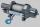How many meters of rope 10 mm thick will fit on the bobbin diameter of 200 mm and length 350 mm (central mandrel have a diameter 50 mm)?
• LadderAdam placed the ladder of the house, the upper end reaching to the window at the height of 3.6m, and the lower end standing on level ground and was distant from a wall of 1.5m. What is the length of the ladder?
• SquareCalculate the area of the square shape of the isosceles triangle with the arms 50m and the base 60m. How many tiles are used to pave the square if the area of one tile is 25 dm2?
• TrapezoidArea of trapezoid is 135 cm2. Sides a, c and height h are in a ratio 6:4:3. How long are a,c and h? Make calculation...
• Church roof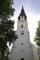The roof of the church tower has the shape of a regular tetrahedral pyramid with base edge length 5.4 meters and a height 5 m. It was found that needs to be corrected 27% covering of the roof area. What amount of material will be required?
• The triangles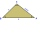The triangles KLM and ABC are given, which are similar to each other. Calculate the lengths of the remaining sides of the triangle KLM, if the lengths of the sides are a = 7 b = 5.6 c = 4.9 k = 5
• EmbankmentPerpendicular cross-section of the embankment around the lake has the shape of an isosceles trapezoid. Calculate the perpendicular cross-section, where bank is 4 m high the upper width is 7 m and the legs are 10 m long.
• Lateral surface areaThe ratio of the area of the base of the rotary cone to its lateral surface area is 3: 5. Calculate the surface and volume of the cone, if its height v = 4 cm.
• ChordIt is given to a circle k(r=6 cm) and the points A, B such that / AB / = 8 cm lies on k. Calculate the distance of the center of circle S to the midpoint C of the segment AB.
• Overload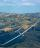Calculate how many g's (gravity accelerations) feel glider pilot when turning the horizontal circles of radius 148 m flying at 95 km/h. Centripetal acceleration is proportional to the square of the speed and inversely proportional to the radius of rotatio
• Rectangular trapezoidIn a rectangular trapezoid ABCD with right angles at vertices A and D with sides a = 12cm, b = 13cm, c = 7cm. Find the angles beta and gamma and height v.
• Cuboid edges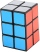The lengths of the cuboid edges are in the ratio 2: 3: 4. Find their length if you know that the surface of the cuboid is 468 m2.
• Picture frames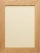Destiny has to make 24 picture frames. Each frame uses 16 3/8 wood trim. 2/3 were completed on the first day. How many inches of wood trim is needed to complete the remaining frames on the second day?
• The diagram 2The diagram shows a cone with slant height 10.5cm. If the curved surface area of the cone is 115.5 cm2. Calculate correct to 3 significant figures: *Base Radius *Height *Volume of the cone
• Area and two anglesCalculate the size of all sides and internal angles of a triangle ABC, if it is given by area S = 501.9; and two internal angles α = 15°28' and β = 45°.
• CincinnatiA map is placed on a coordinate grid. Cincinnati located at (5,4) and San Diego is located at (-10, -3). How far apart is Cincinnati from San Diego on the map? Round to the nearest tenth.
• Circle and rectangleA rectangle with sides of 11.7 cm and 175 mm is described by circle. What is its length? Calculate the content area of the circle described by this circle.
• Tetrahedral pyramidDetermine the surface of a regular tetrahedral pyramid when its volume is V = 120 and the angle of the sidewall with the base plane is α = 42° 30'.
• Metal sheet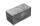How much m ^ 2 of sheet metal is needed to beat a box in the shape of a cube with an edge length of 50 cm, if we add 20% on the folds of the lid and walls?
• Cuboid easyThe cuboid has the dimensions a = 12 cm, b = 9 cm, c = 36 cm. Calculate the length of the body diagonal of the cuboid.

Do you have an interesting mathematical word problem that you can't solve it? Submit a math problem, and we can try to solve it.

We will send a solution to your e-mail address. Solved examples are also published here. Please enter the e-mail correctly and check whether you don't have a full mailbox.

Please do not submit problems from current active competitions such as Mathematical Olympiad, correspondence seminars etc...

See also more information on Wikipedia.